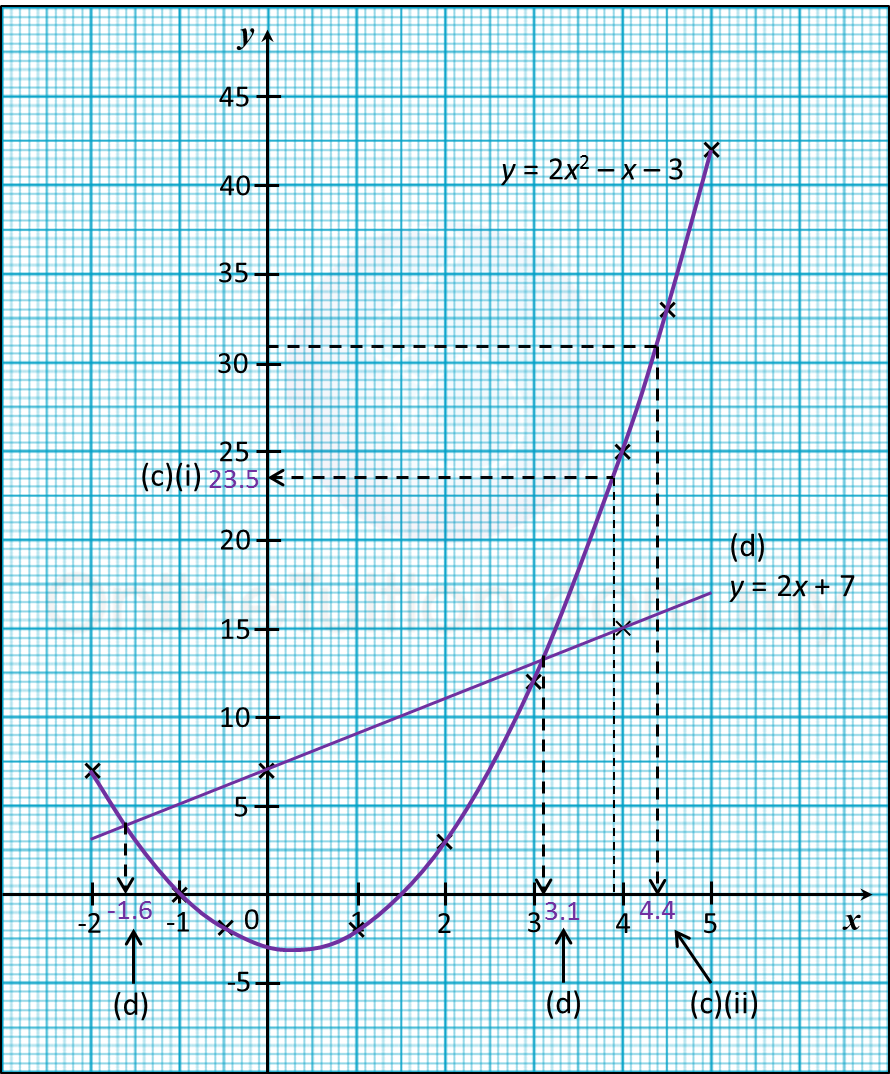# 2.2 Solution of an Equation by Graphical Method

2.2 Solving equations graphically

The solution of the equation f (x) = g (x) can be solved by graphical method.

Step 1
: Draw the graphs of y = f (x) and y = g (x) on the same axes.
Step 2: The points of intersection of the graphs are the solutions of the equation f (x) = g(x). Read the values of x from the graph.

Solution of an Equation by Graphical Method

Example 1:
(a) The following table shows the corresponding values of x and y for the equation = 2x2 x – 3.

 x –2 –1 –0.5 1 2 3 4 4.5 5 y 7 m – 2 –2 3 12 n 33 42
Calculate the value of m and n.

(b) For this part of the question, use graph paper. You may use a flexible curve rule.
By using a scale of 2 cm to 1 unit on the x-axis and 2 cm to 5 units on the y-axis, draw the graph of  y = 2x2 x – 3 for –2 ≤ x ≤ 5.

(c)
(iThe value of when x = 3.9,
(iiThe value of when y = 31.

(d) Draw a suitable straight line on your graph to find the values of x which satisfy the equation 2x 3x = 10 for –2 ≤ x ≤ 5.

Solution:
(a)
= 2x2 x – 3
when x = –1,
= 2 (–1)2 – (–1) – 3 = 0
when x = 4,
= 2 (4)2 – (4) – 3 = 25

(b)(c)
(i) From the graph, when x = 3.9, y = 23.5
(iiFrom the graph, when y = 31, x = 4.4

(d)
= 2x2 x – 3 —– (1)
2x 3x = 10 —– (2)
= 2x2 x – 3 —– (1)
0 = 2x2 3x – 10 —— (2) ← (Rearrange (2))
(1)  – (2) : y = 2x + 7
The suitable straight line is y = 2x + 7.

Determine the x-coordinates of the two points of intersection of the curve
y = 2x2 x – 3 and the straight line y = 2x+ 7.

 x 0 4 y = 2x + 7 7 15
From the graph, = –1.6, 3.1